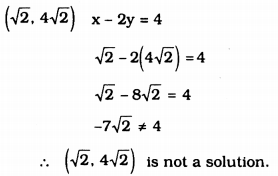# KSEEB Solutions for Class 9 Maths Chapter 10 Linear Equations in Two Variables Ex 10.2

KSEEB Solutions for Class 9 Maths Chapter 10 Linear Equations in Two Variables Ex 10.2 are part of KSEEB Solutions for Class 9 Maths. Here we have given Karnataka Board Class 9 Maths Chapter 10 Linear Equations in Two Variables Exercise 10.2.

## Karnataka Board Class 9 Maths Chapter 10 Linear Equations in Two Variables Ex 10.2

Question 1.
Which one of the following options is true, and why ?
y = 3x + 5 has
i) a unique solution,
ii) only two solutions,
iii) infinitely many solutions.
Solution:
y = 3x + 5 has many solutions. Because this is a linear equation with two variables.

Question 2.
Write four solutions for each of the following equations :
i) 2x + y = 7
ii) πx + y = 9
iii) x = 4y
Solution:
i) 2x + y = 7
If x = 0, 2x + y = 7
2 × 0 + y = 7
0 + y = 7
y = 7
Solution is (0, 7).
If x = 1, 2x + y = 7
2 × 1 + y = 7
2 + y = 7
y = 7 – 2 = 5
Solution is (1, 5).
If x = 2, 2x + y = 7
2 × 2 + y = 7
4 + y = 7
y = 7 – 4 = 3
Solution is (2, 3).
If x = 3, 2x + y = 7
2 × 3 + y = 7
6 + y = 7
∴ y = 7 – 6 = 1
Solution is (3, 1).

ii) πx + y = 9
If x = 0, πx + y = 9
π × 0 + y = 9
0 + y = 9
y = 9
Solution (x, y) = (0, 0).
If x = 1, πx + y = 9
π × 1 + y = 9
π + y = 9
y = 9 – π
Solution (x, y) = (1, 9 – π).
If y = 0, πx + y = 9
πx + 0 = 9
πx = 9
∴ x = $$\frac{9}{\pi}$$
Solution (x, y) = ( $$\frac{9}{\pi}$$, o).
If y = 1, πx + y = 9
πx + 1 = 9
πx = 9 – 1
πx = 8
∴ x = $$\frac{8}{\pi}$$
Solution (x, y) = ($$\frac{8}{\pi}$$ ,1).

iii) x = 4y
If x = 0, x = 4y
0 = 4y
4y = 0
∴ y = $$\frac{0}{4}$$ = ∞
∴ Solution (x, y) = (0, ∞).
If x = 1, x = 4y
1 = 4y
4y = 1
∴ y = $$\frac{1}{4}$$
∴ Solution (x, y) = (1, $$\frac{1}{4}$$).
If x = 2, x = 4y
2 = 4y
4y = 2
∴ y = $$\frac{4}{2}$$
∴ y = $$\frac{1}{2}$$
Solution (x, y) = (2, $$\frac{1}{2}$$).
If x = 4, x = 4y
4 = 4y
4y = 4
∴ y = $$\frac{4}{4}$$
∴ y = 1
∴ Solution (x, y) = (4, 1).

Question 3.
Check which of the following are solutions of the equation x – 2y = 4 and which are not:
i) (0, 2)
ii) (2, 0)
iii) (4, 0)
iv) ($$\sqrt{2}, 4 \sqrt{2}$$)
v) (1, 1)
Solution:
i) (0, 2)
x – 2y = 4
0 – 2(2) = 4
0 – 4 = 4
-4 ≠ 4
∴ (0, 2) is not a solution.

ii) (2, 0)
x – 2y = 4
2 – 2(0) = 4
2 – 0 = 4
2 ≠ 4
∴ (2, 0) is not a solution

iii) (4, 0)
x – 2y = 4
4 – 2(0) = 4
4 – 0 = 4
4 = 4
∴ (4, 0) is a solution.

iv)v) (1, 1)
x – 2y = 4
1 – 2(1) = 4
1 – 2 = 4
-1 ≠ 4
∴ (1, 1) is not a solution.

Question 4.
Find the value of k, if x = 2, y = 1 is a solution of the equation 2x + 3y = k.
Solution:
2x + 3y = k
If x = 2, y = 1, then k = ?
2x + 3y = k
2(2) + 3(1) = k
4 + 3 = k
7 = k
∴ k = 7.

We hope the KSEEB Solutions for Class 9 Maths Chapter Linear Equations in Two Variables Ex 10.2 help you. If you have any query regarding Karnataka Board Class 9 Maths Chapter Linear Equations in Two Variables Exercise 10.2, drop a comment below and we will get back to you at the earliest.